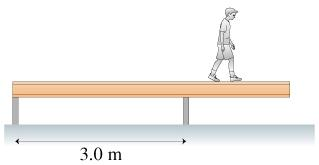# Problem: A 37 kg , 5.0-m-long beam is supported, but not attached to, the two posts in the figure. A 20 kg boy starts walking along the beam. How close can he get to the right end of the beam without it falling over?

###### FREE Expert Solution

The conditions for static equilibrium:

$\overline{){\mathbf{\Sigma }}{\mathbf{F}}{\mathbf{=}}{\mathbf{0}}}$

and

$\overline{){\mathbf{\Sigma }}{\mathbf{\tau }}{\mathbf{=}}{\mathbf{0}}}$

Let d be the distance from the second post the boy walks without the beam falling.

The distance between the center of the beam and the second post is (3.0-2.5 = 0.50m). M is the mass of the beam, and m is the mass of the boy.

100% (267 ratings)###### Problem DetailsA 37 kg , 5.0-m-long beam is supported, but not attached to, the two posts in the figure. A 20 kg boy starts walking along the beam.

How close can he get to the right end of the beam without it falling over?